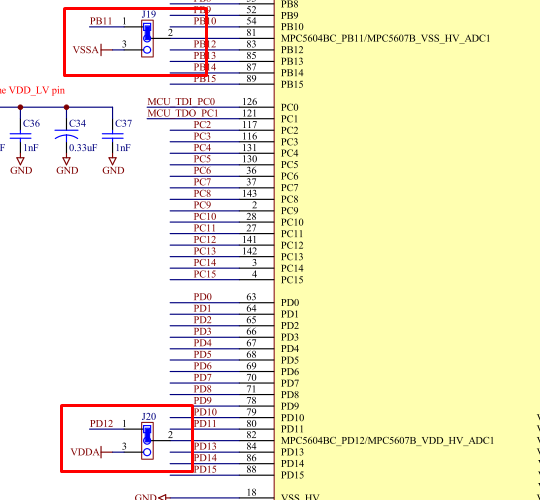713 次查看Contributor III

I am Trying to run this code in which, Potentiometer is connected to PB -> ADC1_P  (ADC_1) and Its output is Shown on LED1,2,3,4.  I am trying to run ADC1 in scan mode but attached program is not working properly.

I tried to similar code for ADC_0 (scan mode) and it was working perfectly (showing as expected output).

From the comments in the program, You can see the ADC_0 code.

Connections:

Potentiometer is connected to PB -> ADC1_P  (ADC_1) and Its output is Shown on LED1,2,3,4 connected to PE, PE, PE and PE respectively.

My ADC_1 (Scan mode) is as shown below:

*********************************************************************************************************************************************

/* ADC0_ScanMoode example for MPC5606B (Tested on TRK-MPC5606B Board)

Description: Converts inputs ANS0, ANS1 and ANS2 using scan mode (continuous)

Program Outcome:

(PB)   [LED1 LED2 LED3 LED4]

DigitalValue (0-200) => ON OFF OFF OFF

DigitalValue (201-500) => ON ON OFF OFF

DigitalValue (501-800) => ON ON ON OFF

DigitalValue (801-1023) => ON ON ON ON

*/

/*Connections:

100 144  176

ANS0 CH32 B8 PCR=2000 39 53  R9

ANS1 CH33 B9 PCR=2000 38 52  T9

ANS2 CH34 B10 PCR=2000 40 54   P9

ANP0 CH0 B4 PCR=2000 50   72   88

ANP1 CH1 B5 PCR=2000 53   73   91

ANP2 CH2 B6 PCR=2000 54   76   92

NOTE: Do not forget to add "ME.PCTL.R = 0x01;" line

in void initModesAndClock(void) function to make ADC1 work.

*/

#include "MPC5606B.h"

#define GPIO_INPUT 0x0101

#define GPIO_OUTPUT 0x0201

#define LED_ON 0

#define LED_OFF 1

#define LED1 68

#define LED2 69

#define LED3 70

#define LED4 71

void initModesAndClock(void);

void disableWatchdog(void);

void initPeriClkGen(void);

void main (void);

uint16_t Result; // ADC conversion results

uint16_t ResultInMv; // ADC conversion results in mv

void initModesAndClock(void) {

ME.MER.R = 0x0000001D; /* Enable DRUN, RUN0, SAFE, RESET modes */

/* Initialize PLL before turning it on: */

CGM.FMPLL_CR.R = 0x02400100; /* 8 MHz xtal: Set PLL0 to 64 MHz */

ME.RUN.R = 0x001F0074; /* RUN0 cfg: 16MHzIRCON,OSC0ON,PLL0ON,syclk=PLL0*/

ME.RUNPC.R = 0x00000010; /* Peri. Cfg. 1 settings: only run in RUN0 mode */

ME.PCTL.R = 0x01; /* MPC56xxB/P/S ADC 0: select ME.RUNPC */

ME.PCTL.R = 0x01; //ADC 1: Select ME.RUNPC

ME.PCTL.R = 0x01; /* MPC56xxB/S SIU: select ME.RUNPC */

/* Mode Transition to enter RUN0 mode: */

ME.MCTL.R = 0x40005AF0; /* Enter RUN0 Mode & Key */

ME.MCTL.R = 0x4000A50F; /* Enter RUN0 Mode & Inverted Key */

while (ME.GS.B.S_MTRANS) {} /* Wait for mode transition to complete */

/* Note: could wait here using timer and/or I_TC IRQ */

while(ME.GS.B.S_CURRENTMODE != 4) {} /* Verify RUN0 is the current mode */

}

void disableWatchdog(void) {

SWT.SR.R = 0x0000c520; /* Write keys to clear soft lock bit */

SWT.SR.R = 0x0000d928;

SWT.CR.R = 0x8000010A; /* Clear watchdog enable (WEN) */

}

void initPeriClkGen(void) {

CGM.SC_DC0.R = 0x80; /* MPC56xxB/S: Enable peri set 3 sysclk divided by 1 */

CGM.SC_DC1.R = 0x80; /* MPC56xxB/S: Enable peri set 3 sysclk divided by 1 */

CGM.SC_DC2.R = 0x80; /* MPC56xxB/S: Enable peri set 3 sysclk divided by 1 */

}

void main (void) {

vuint32_t i = 0; /* Dummy idle counter */

initModesAndClock(); /* Initialize mode entries and system clock */

disableWatchdog(); /* Disable watchdog */

initPeriClkGen(); /* Initialize peripheral clock generation for DSPIs */

//SIU.PCR.R = 0x2000; /* MPC56xxS: Initialize PB as ANS0 */

//SIU.PCR.R = 0x2000; /* MPC56xxS: Initialize PB as ANS1 */

//SIU.PCR.R = 0x2000; /* MPC56xxS: Initialize PB as ANS2 */

SIU.PCR.R = 0x2000; //Initialize PB as ANP0 (ADC1)

SIU.PCR.R = 0x2000; //Initialize PB as ANP1 (ADC1)

SIU.PCR.R = 0x2000; //Initialize PB as ANP2 (ADC1)

SIU.PCR[LED1].R = GPIO_OUTPUT;  //GPIO as Output //LED1

SIU.PCR[LED2].R = GPIO_OUTPUT;  //GPIO as Output //LED2

SIU.PCR[LED3].R = GPIO_OUTPUT;  //GPIO as Output //LED3

SIU.PCR[LED4].R = GPIO_OUTPUT;  //GPIO as Output //LED4

//ADC_0.MCR.B.PWDN = 0; //Power Down mode Disabled

ADC_1.MCR.B.PWDN = 0; //Power Down mode Disabled

//ADC_0.NCMR1.R = 0x00000007; // Select ANS0:2 inputs for conversion

//ADC_0.NCMR1.B.CH32 = 1; //Select ANS0 input for Conversion

//ADC_0.NCMR1.B.CH33 = 1; //Select ANS1 input for Conversion

//ADC_0.NCMR1.B.CH34 = 1; //Select ANS2 input for Conversion

ADC_1.CTR0.R = 0x00008606; // Set Conversion time

SIU.GPDO[LED1].B.PDO = LED_OFF;

SIU.GPDO[LED2].B.PDO = LED_OFF;

SIU.GPDO[LED3].B.PDO = LED_OFF;

SIU.GPDO[LED4].B.PDO = LED_OFF;

while (1) {

while (ADC_1.CDR.B.VALID != 1) {}; /* Wait for last scan to complete */

//ResultInMv = (uint16_t) (5000*Result/0x3FF); /* Converted result in mv */

//ResultInMv = (uint16_t) (5000*Result/0x3FF); /* Converted result in mv */

//ResultInMv = (uint16_t) (5000*Result/0x3FF); /* Converted result in mv */

if(Result<=1000){

SIU.GPDO[LED1].B.PDO = LED_ON;

SIU.GPDO[LED2].B.PDO = LED_OFF;

SIU.GPDO[LED3].B.PDO = LED_OFF;

SIU.GPDO[LED4].B.PDO = LED_OFF;

}

else if(Result>1000 && Result<=2000){

SIU.GPDO[LED1].B.PDO = LED_ON;

SIU.GPDO[LED2].B.PDO = LED_ON;

SIU.GPDO[LED3].B.PDO = LED_OFF;

SIU.GPDO[LED4].B.PDO = LED_OFF;

}

else if(Result>2000 && Result<=3000){

SIU.GPDO[LED1].B.PDO = LED_ON;

SIU.GPDO[LED2].B.PDO = LED_ON;

SIU.GPDO[LED3].B.PDO = LED_ON;

SIU.GPDO[LED4].B.PDO = LED_OFF;

}

else if(Result>3000 && Result<=4095){

SIU.GPDO[LED1].B.PDO = LED_ON;

SIU.GPDO[LED2].B.PDO = LED_ON;

SIU.GPDO[LED3].B.PDO = LED_ON;

SIU.GPDO[LED4].B.PDO = LED_ON;

}

i++;

}

}

*********************************************************************************************************************************************

Original Attachment has been moved to: ADC1_ScanMode_APDaga.rar

• ### General

1 解答
438 次查看NXP TechSupport

Hi,

Your code works properly. You only have to supply the ADC1 reference. Jumpers J19 and J20 are used for that purpose.

So place both  jumpers to position 2-3.BR, Petr

2 回复数
439 次查看NXP TechSupport

Hi,

Your code works properly. You only have to supply the ADC1 reference. Jumpers J19 and J20 are used for that purpose.

So place both  jumpers to position 2-3.BR, Petr

438 次查看Contributor III

Thank you Petr Stancik.
It worked after connecting Jumpers J19 and J20 on TRK-MPC5606B Board.